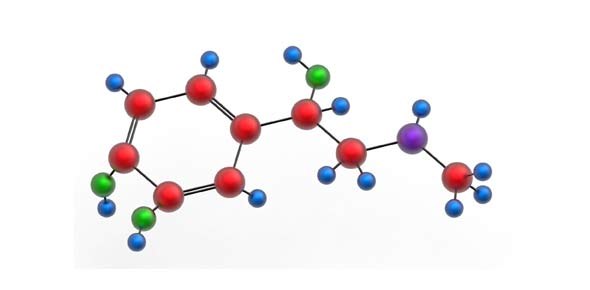# Formula Of Ionic Compounds Quiz

20 Questions | Attempts: 1298SettingsAnswer the following questions based on your knowledge of ionic compounds. Let's start this awesome quiz now!

• 1.
What is the formula for table salt?
• A.

Na1Cl1

• B.

Na2Cl2

• C.

Na2Cl1

• D.

Na1Cl2

• 2.
What is the formula for Aluminum oxide?
• A.

AlO

• B.

Al2O3

• C.

Al3O2

• D.

Al2O2

• 3.
What is the oxidation number on the phosphorus atom 'P' in chromium (III) phosphate, Cr(PO4)
• A.

-2

• B.

+3

• C.

+4

• D.

+5

• 4.
The cation is always written first in an ionic compound.
• A.

True

• B.

False

• 5.
Ammonium nitrate is used as a fertilizer, to make cold packs, and as an explosive. What is the formula of ammonium nitrate?
• A.

(NH)(NO)

• B.

(NH3)(NO3)

• C.

(NH4)(NO3)

• D.

(NH4)(NO2)

• 6.
Cations are atoms that have _____________ electrons.
• 7.
What is the charge on any ion of an alkali metal?
• A.

+1

• B.

+2

• C.

-1

• D.

-2

• 8.
What is the charge on the ion of any halogen?
• A.

+1

• B.

+2

• C.

-1

• D.

-2

• 9.
What is the traditional name for the following ionic compound...  FeO?
• A.

Ferrous oxide

• B.

Ferric oxide

• C.

Ferrum oxide

• D.

Ferrylicious oxidocious

• 10.
What is the stock name given to the following ionic compound...  CuCl2?
• A.

Copper (I) chloride

• B.

Copper (II) chloride

• C.

Copper (III) chloride

• D.

Cupric chloride

• 11.
The number (+2) when written in a formula of an ion would be written as a subscript.
• A.

True

• B.

False

• 12.
What is the charge on the lead ion (Pb) in the following formula...  PbO?
• A.

+1

• B.

+2

• C.

+3

• D.

+4

• 13.
What is the stock name for the ionic compound with the formula of...  Cu(NO3)
• A.

Copper (II) nitrate

• B.

Copper (II) nitrite

• C.

Copper (I) nitrate

• D.

Copper nitride

• 14.
What is the correct formula for the ionic compound made from the following ions...  Ba+2 and (HClO)-1
• A.

Ba2(HClO)

• B.

Ba2(HClO)3

• C.

Ba(HClO)

• D.

Ba(HClO)2

• 15.
An ion that is made up of morr that one atom is called a ____________ ion.
• 16.
What would be the correct 'acid name' for the following binary acid;  HBr, chemical name of hydrogen bromide
• A.

Hydrogen bronide

• B.

Hydrobromic acid

• C.

Hydrogen bromic acid

• D.

Bromic acid

• 17.
What is the 'acid' name of the following ternary acid;  HClO, chemical name hydrogen hypochlorite
• A.

Hypochlorous acid

• B.

Hydrochlorous acid

• C.

Hydrogen chlorous acid

• D.

Hypochloric acid

• 18.
A compound formed between ions that have gained and lost electrons is called a(n) ___________ compound.
• 19.
How many total ions are in the following ionic compound formula...  Na2(SO4)1
• A.

3

• B.

2

• C.

1

• D.

7

• 20.
The formula for ionic compounds must be written using the lowest whole number ratio betwen ions.
• A.

True

• B.

False

## Related TopicsBack to top
×

Wait!
Here's an interesting quiz for you.